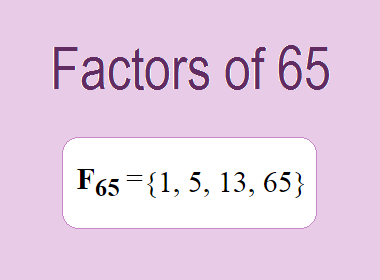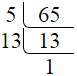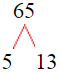# Factors of 65The factors of 65 are 1, 5, 13, and 65 i.e. F65 = {1, 5, 13, 65}. The factors of 65 are all the numbers that can divide 65 without leaving a remainder.

We can check if these numbers are factors of 65 by dividing 65 by each of them. If the result is a whole number, then the number is a factor of 65. Let's do this for each of the numbers listed above:

·        1 is a factor of 65 because 65 divided by 1 is 65.

·        5 is a factor of 65 because 65 divided by 5 is 13.

·        13 is a factor of 65 because 65 divided by 13 is 5.

·        65 is a factor of 65 because 65 divided by 65 is 1.

## How to Find Factors of 65?

1 and the number itself are the factors of every number. So, 1 and 65 are two factors of 65. To find the other factors of 65, we can start by dividing 65 by the numbers between 1 and 65. If we divide 65 by 2, we get a remainder of 1. Therefore, 2 is not a factor of 65. If we divide 65 by 3, we get a remainder of 2. Therefore, 3 is not factor of 65.

Next, we can check if 4 is a factor of 65. If we divide 65 by 4, we get a remainder of 1. Therefore, 4 is not a factor of 65. If we divide 65 by 5, we get a remainder of 0. Therefore, 5 is a factor of 65. We can continue this process for all the possible factors of 65.

Through this process, we can find that the factors of 65 are 1, 5, 13, and 65. These are the only numbers that can divide 65 without leaving a remainder.

********************

********************

## Properties of the Factors of 65

The factors of 65 have some interesting properties. One of the properties is that the sum of the factors of 65 is equal to 84. We can see this by adding all the factors of 65 together:

1 + 5 + 13 + 65 = 84

Another property of the factors of 65 is that they are all odd numbers. This is because 65 is an odd number, and any even number cannot divide an odd number without leaving a remainder.

Another property of the factors of 65 is that the prime factors of 65 are 5 and 13 only.

## Applications of the Factors of 65

The factors of 65 have several applications in mathematics. One of the applications is in finding the highest common factor (HCF) of two or more numbers. The HCF is the largest factor that two or more numbers have in common. For example, to find the HCF of 65 and 52, we need to find the factors of both numbers and identify the largest factor they have in common. The factors of 65 are 1, 5, 13, and 65. The factors of 52 are 1, 2, 13, 26, and 52. The largest factor that they have in common is 13. Therefore, the HCF of 65 and 52 is 13.

Another application of the factors of 65 is in prime factorization. Prime factorization is the process of expressing a number as the product of its prime factors. The prime factors of 65 are 5 and 13. We can express 65 as:

65 = 5 × 13

We can do prime factorization by division and factor tree method also. Here is the prime factorization of 65 by division method,65 = 5 × 13

Here is the prime factorization of 65 by the factor tree method,65 = 5 × 13

## Conclusion

The factors of 65 are the numbers that can divide 65 without leaving a remainder. The factors of 65 are 1, 5, 13, and 65. The factors of 65 have some interesting properties, such as being odd numbers and having a sum of 84. The factors of 65 have several applications in mathematics, such as finding the highest common factor and prime factorization.